# How to Draw a Two Point Perspective Grid Tutorial

## Two Point Perspective Grid Tutorial | Video Transcript

Today I’m going to show you how to draw a two point perspective grid and some illustration examples.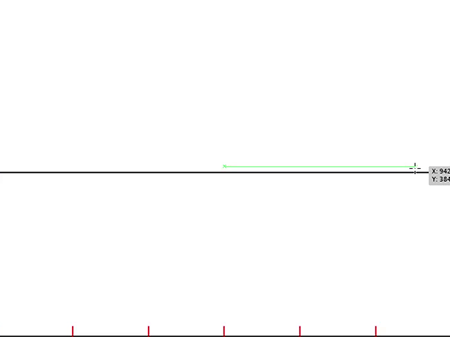Step 1. Draw a horizon line across the page. At the bottom of the page, add several evenly distributed markers, as seen in red.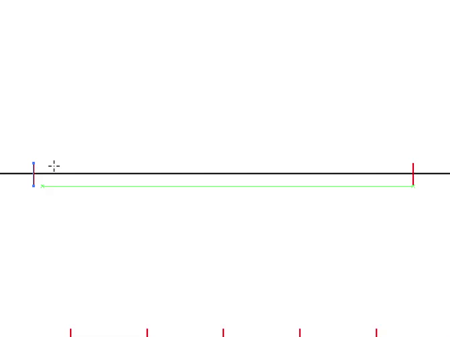Step 2. Mark 2 vanishing points. Their location on the horizon line is chosen arbitrarily.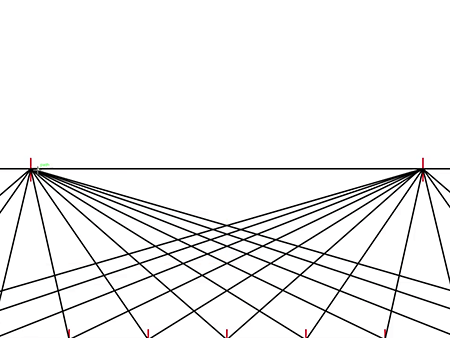Step 3. Draw lines from the vanishing points to the red markers at the bottom of the page. Some of the red markers extend beyond the page in order to draw a full perspective grid. Your basic grid is now complete.

## Examples

Now I’ll show you some perspective drawing examples.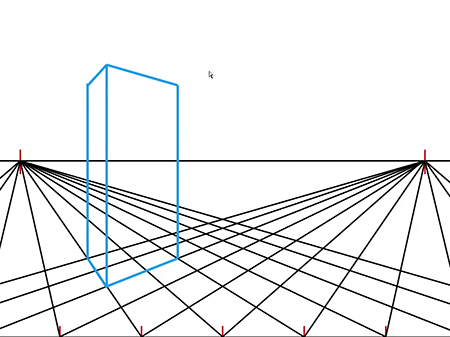Example 1: This is how you draw a cube that starts below the horizon line and ends above the horizon line. Notice how you can see neither the top nor bottom surface of the shape.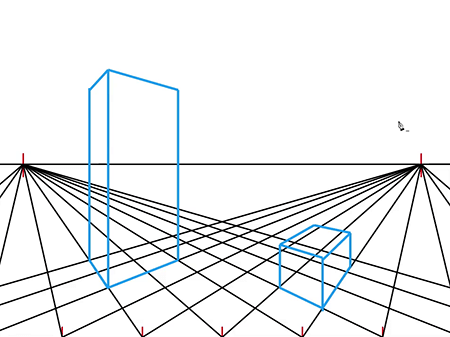Example 2: This is how you draw a cube that starts and ends below the horizon line. Notice how you can see the top surface of the shape.Example 3: This is how you draw a cube that starts and finishes above the horizon line. Notice how you can see the bottom surface of the shape.

Example 4: This example will show you how to correctly illustrate foreshortening along a path leading toward the horizon. This is useful when drawing pillars, trees along a road, or a line of people.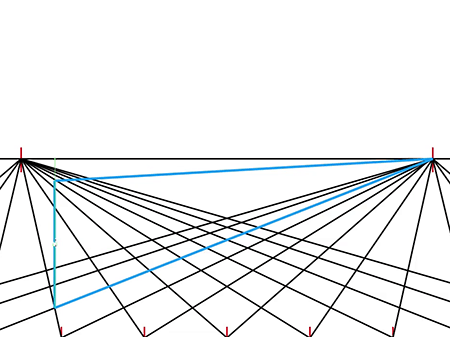Step 1: Draw a box extending to the horizon.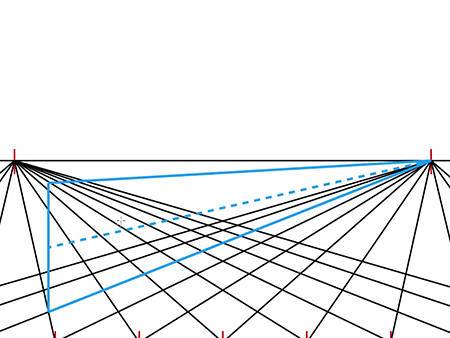Step 2: Draw a line down the middle of the box.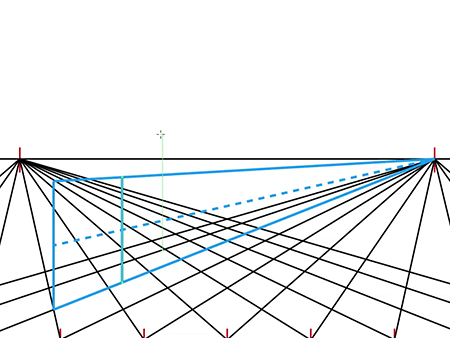Step 3: Draw a vertical line sectioning off the box. Inside this new box is where you would draw the trees, pillars, people etc.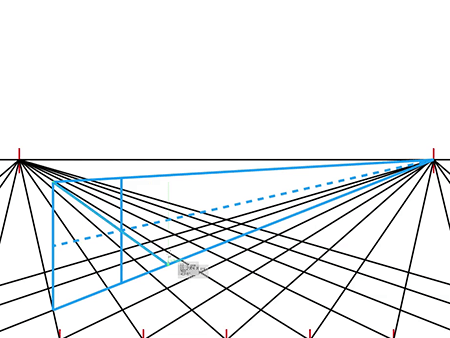Step 4: Draw a diagonal from the top left corner of the box through the point where the center line and the second vertical line intersect. Continue this diagonal until it hits the bottom of the box extending to the vanishing point. Draw another vertical line.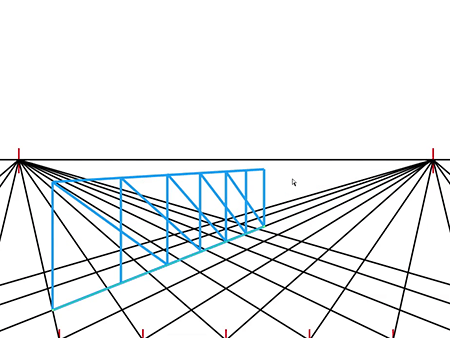Step 5: Repeat the process until you have enough boxes.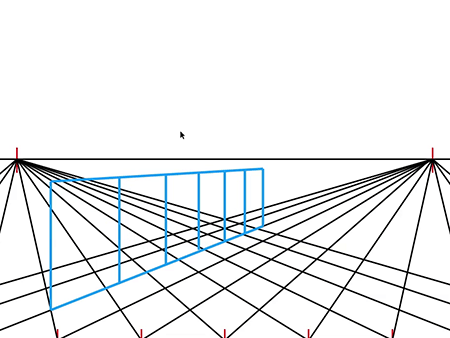Erase any unwanted lines. Notice how the boxes become skinnier. This is due to foreshortening.

### 1 thought on “How to Draw a Two Point Perspective Grid Tutorial”

1.Hi,

Thank you for the tutorial, very helpful! Can you explain how do we establish the height of the object? Let’s say, I would like to create 2 boxes; one is 1×2 and the second one is 2×2?

Thanks, Karolina